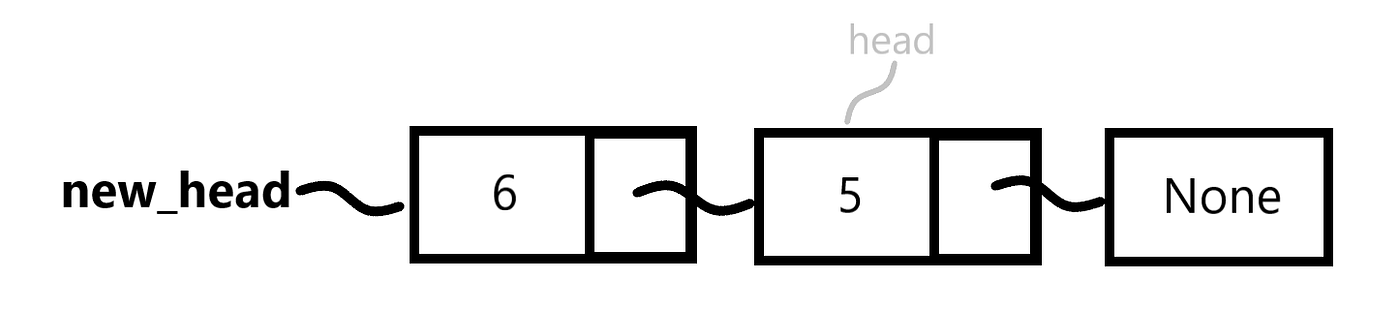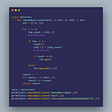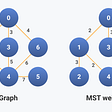# How to recursively reverse a linked list

## An intuition-building approach

Before discussing the recursive algorithm, it’s important for us to understand why we are able to use recursion in the first place.

Here’s the big idea. The linked list is a recursive data structure, which means we can use recursive functions to query or perform operations on it.

We can use recursive functions to query or perform operations on recursive data structures.

## Hold up! What’s a recursive data structure?

Typically, we learn that some functions can be defined recursively. These functions are called recursive functions.

A recursive function is a function that calls itself.

The idea that some data structures can be defined recursively doesn’t get nearly as much attention.

A recursive data structure is made up of data structures of the same type.

The linked list data structure is recursive, which tells us we can use recursive algorithms to query or perform operations on it.

Is this idea of a recursive data structure still unclear?

Take a look at how we define the `LinkedListNode` class below.

Notice that to create a node, we need to assign a node to `self.next_node`. Doesn’t that sound or look like recursion?

Similar to how we need a function to be called within itself for the function to be classified as recursive, we can classify a data structure as recursive if the data structure is made up of data structures of the same type.

You’re probably wondering about the case when `next_node` is `None`. This might sound a little weird, but in this case, we can think of `None` as a `LinkedListNode` in the same way zero is a number.

Think about it like this. `None` is a `LinkedListNode` that represents the absence of a node, similar to how zero represents the absence of the stuff we’re interested in counting.

Another way to think about it is that `None` for `self.next_node` is the “base case” for the recursive data structure. In this sense, it indicates that we’ve reached the end of the linked list.

Concrete examples of recursive data structures go beyond linked lists. The popular tree and graph data structures are recursive too.

## Recursive linked list reversal algorithm

As explained in the previous section, we can use a recursive function to perform operations on recursive data structures. Let us reverse a linked list, starting with an empty list and incrementally solving more complex cases.

How do we handle an empty linked list?

How do we handle a linked list with one node?

We’re returning the same thing in both cases so we could collapse these two if statements into a single if statement with a compound condition like so.

However, we’ll keep using the previous version for maximum clarity. More importantly, these if statements form the base cases of the recursive function we’re building.

How do we handle a linked list with two nodes?Diagram of a 2-node linked list. First node has value 5. Second node has value 6.

Take a moment to walk through this code before we make it recursive. There’s nothing fancy here, just a swap of the first and last nodes in the linked list. In a 2-node linked list, doing this swap alone successfully reverses the linked list.

However, the function we have now only works for linked lists that are have 0, 1, or 2 nodes. Let’s make the function recursive, but maintain the same behavior of reversing the 0-, 1-, and 2-node linked list.

How do we make this 0-1-2 solution recursive?

With this, we’ve made our linked list reversal function recursive. We changed two lines — the ones following comments A and B— to make the function recursive, but we’re still doing just doing the same swapping we were doing in the previous solution.

Let us examine what happens when a linked list with two nodes is given our recursive function.

First, the two if statements are skipped because we have a list that has more than 0 and 1 nodes.

Next, on the line following comment A, we pass the second node (`head.next_node`) to our recursive function. In a list of two nodes, the second node is our end node, so our recursive function runs into our second if statement and returns the same node.

In effect, the line after comment A in both versions of the code (shown below) produce the same result when dealing with a 2-node linked list.

`# v1new_head = head.next_node# v2new_head = reverse_linked_list_recursive(head.next_node)`

They both label the linked list’s end node as the new head node, which just happens to be the second node in our 2-node linked list.

Visually, this what we have done so far— added a label called `new_head` for the end node in the linked list.

Next, on the line following comment B, we make the new head’s next_node point the old head of the linked list, like so.

`head.next_node.next_node = head`

Just by looking at the image above we can see how the above line of code is equivalent to line of code below from the non-recursive function for reversing a 2-node linked list.

`new_head.next = head`

We make this change because the code above is fragile. It only works for the 2-node linked list. While `head.next_node.next_node` code is equivalent for the 2-node linked list, it also generalizes to other cases as you will soon come to understand.

Visually, this what we have now.

Next, we make `head.next = None` to indicate that head labels the node at the end of the linked this. This is what our linked list loos like.

If we clean things up a bit and focus on the flow from the `new_head`, our linked list actually looks more like this.Diagram of cleaned up and reversed 2-node linked list. First node has value 6. Second node as value 5.

Finally, we return `new_head`.

Does our recursive function work for longer linked lists?

Try it! 👨🏿‍💻

The cool thing about recursion is that it often allows us to write short and elegant code by exploiting recursive data structures and the sameness of how we solve sub-problems to solve a larger problem.

We have a recursive function that we know reverses 0-, 1-, and 2-node linked lists. To reverse a 3-node linked list, we can start by holding on to the first node, reverse the remaining 2-nodes using our recursive function, and tack the first node we held to the end of the reversed 2-node sub-linked list.

That’s it. We’ve reversed a 3-node linked list by first solving the sub-problems of reversing a 0-, 1-, and 2-node linked list and reusing the computation to solve a larger problem.

What about the Big-O time and space complexity of this algorithm?

The time complexity for this algorithm is O(n). The algorithm visits all n nodes in the linked list, where n is the number of nodes in the list.

We didn’t create new nodes or any other data structures for that matter. We can tell from the diagrams that all we’ve done is rearrange the links between the linked list’s nodes. However, because this algorithm uses recursion, it implicitly makes use of stack space that grows linearly with the size of the input list, thus the space complexity is O(n).

Thank you for reading! Go forth, and recurse 👨🏿‍💻

Edit: A previous version of this article did not consider the implicit stack space used for recursion and noted the space complexity as O(1). This has been corrected to O(n) to reflect consideration of the stack space used.

--

--

--

## More from The Core

Shared thoughts and ideas about technology

## Using S3 Just Like a Local File System in Python## Breaking Good## Releasing My Algo 2.0: The Most Advanced Algorand Wallet## Architecting Feature Flags For Performance At Scale## PDF Deep linking with AutoHotkey## Onel Harrison

Software Engineer — Data & Machine Learning

## Leetcode 1209 Remove All Adjacent Duplicates in String II## Remove Null, None, and Empty Strings from an n-sub level dictionary## Prims Algorithm